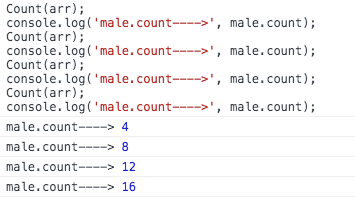## Js中函数式编程

``````const arr = [
{name: '赵信', gender: 1, age: 25, high: 176, weight: 62},
{name: '艾希', gender: 2, age: 23, high: 161, weight: 46},
{name: '阿狸', gender: 2, age: 27, high: 182, weight: 53},
{name: '盖伦', gender: 1, age: 27, high: 175, weight: 78},
{name: '沃里克', gender: 1, age: 42, high: 169, weight: 70},
{name: '安妮', gender: 2, age: 16, high: 153, weight: 43},
{name: '卡尔玛', gender: 2, age: 40, high: 168, weight: 48},
{name: '菲兹', gender: 0, age: 52, high: 163, weight: 50},
{name: '亚索', gender: 1, age: 35, high: 177, weight: 65},
{name: '锐雯', gender: 2, age: 33, high: 172, weight: 52},
]``````

``````const male = {
count: 0,
list: [],
};

const MIN_AGE = 18;

const Count = (arr) => {
for (const item of arr) {
if (
!item
|| +item.age < +MIN_AGE
|| `\${item.gender}` !== '1'
) { continue }
male.count++;
male.list.push({
name: item.name,
high: item.high,
});
}
}``````

1. 不依赖外部状态，相同的输入永远得到相同的输出；

2. 没有副作用，不会修改入参或者全局变量。 // splice 说的就是你！``````const Count = (arr, min) => {
// 创建一个局部变量
const res = {
count: 0,
list: [],
};
for (const item of arr) {
if (
!item
|| +item.age < +min // 使用入参而不是全局变量
|| `\${item.gender}` !== '1'
) { continue }
res.count++;
res.list.push({
name: item.name,
high: item.high,
});
}
// 返回结果
return res;
}``````

``fun(a, b, c)  ->  fun(a)(b)(c)``

``fun(a, b, c) -> fun(a)(b, c) / fun(a, b)(c)``

``const sum = (x, y, z) => x + y + z;``

``````const curry = (fn) => {
return function recursive(...args) {
// 如果args.length >= fn.length则表明传入了足够的参数，此时调用fn并返回
if (args.length >= fn.length) {
return fn(...args);
}

// 否则表明没有传入足够的参数，此时返回一个函数,用这个函数接受后面传递的新参数
return (...newArgs) => {
// 递归调用recursive函数,并返回
return recursive(...args.concat(newArgs));
};
};
};``````

``````const Sum = curry(sum);      // -> [Function]Sum(10)(11)(12);             // -> 33
const Sum10 = Sum(10);       // -> [Function]
const Sum10_11 = Sum10(11);  // -> [Function]
Sum10_11(12);                // -> 33``````

``const compose = (f, g) => x => f(g(x))``

``````const f = x => x + 1;
const g = x => x * 2;
const fg = compose(f, g);
fg(1)  // ----> ?``````

``````filter18(arr);            // 从数组中返回年龄大于18岁的数据
filterMale(arr);          // 从数组中筛选出男性数据并返回新数组
pickNameHeight(arr);      // 获取数组中的姓名和身高字段并返回新数组log(arr);                 // 打印参数``````

``log(pickNameHeight(filterMale(filter18(arr))));``

``````const fun = compose(log, pickNameHeight, filterMale, filter18);
fun(arr);``````

1. 过滤出18岁以上的数据，首先需要实现一个用于比较大小的工具函数

``````// 校验对象中的某个 key 是否大于临界值 val
function porpGt(key, val, item) {    return item[key] > val
}``````

``````const cPropGt = curry(porpGt);        // porpGt(a, b, c) -> cPropGt(a)(b)(c)
const filter18 = cPropGt('age')(18);  // cPropGt('age')(18)(item) -> filter18(item)
arr.filter(filter18);                 // 返回 age 大于 18 的数据``````

2. 过滤出男性，这需要一个判断等值的工具函数

``````// 判断对象中的某个 key 是否等于临界值 val
function porpEq(key, val, item) {
return `\${item[key]}` === `\${val}`
}``````

``````const cPropEq = curry(porpEq);            // porpEq(a, b, c) -> cPropEq(a)(b)(c)
const filterMale = cPropEq('gender')(1);  // cPropEq('gender')(1)(item) -> filterMale(item)
arr.filter(filterMale);                   // 返回 gender 等于 1 的数据``````

3. 记录身高和姓名，需要一个从对象中提取值的工具函数

``````// 从对象中提取多个值并返回新的对象
function pickAll(keys, item) {
const res = {};
keys.map(key => res[key] = item[key]);
return res;
}``````

``````const cPickAll = curry(pickAll);
const pickProps = cPickAll(['name', 'high']);
arr.map(pickProps);   // 只保留 name 和 high``````

``````arr.filter(filter18)
.filter(filterMale)
.map(pickProps)``````

``````const Count = compose(
map(pickProps),
filter(filterMale),
filter(filter18),
);
Count(arr);``````

1.云服务推荐: 国内主流云服务商，各类云产品的最新活动，优惠券领取。地址：阿里云腾讯云华为云

2.广告联盟: 整理了目前主流的广告联盟平台，如果你有流量，可以作为参考选择适合你的平台点击进入